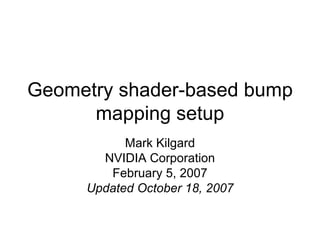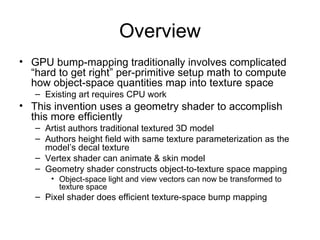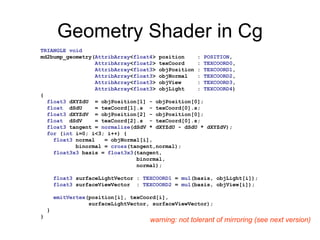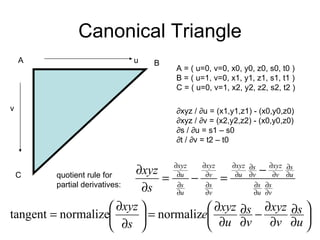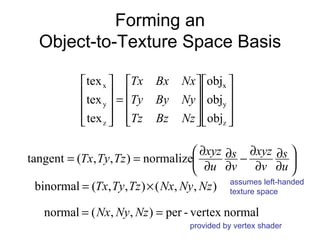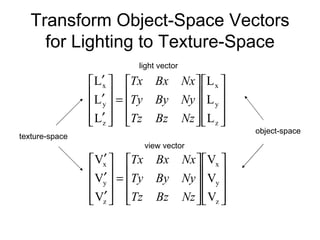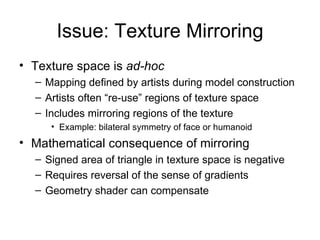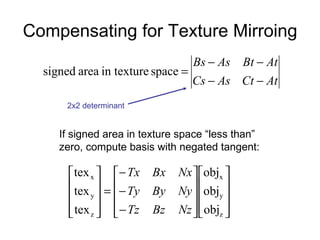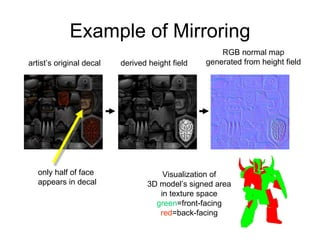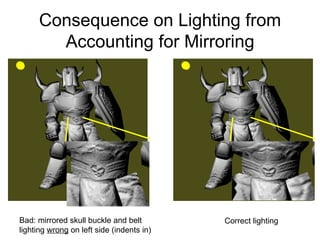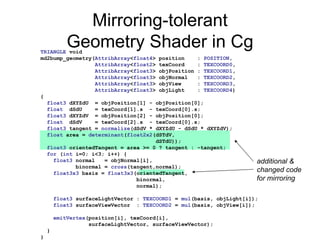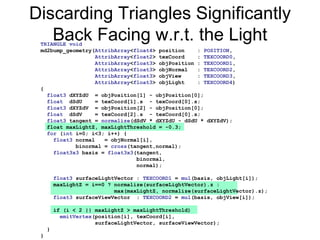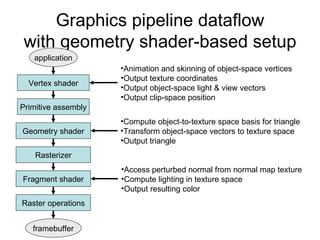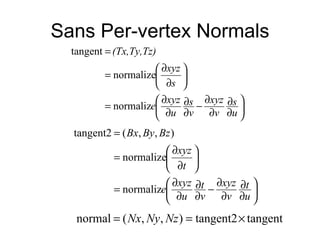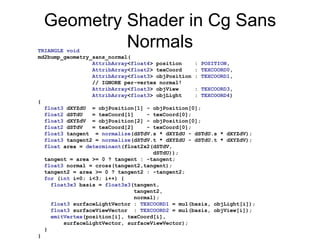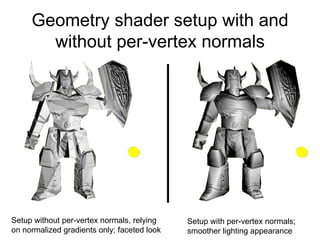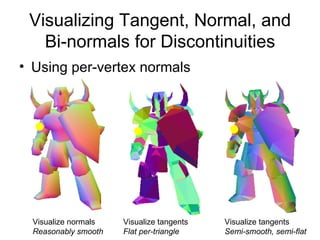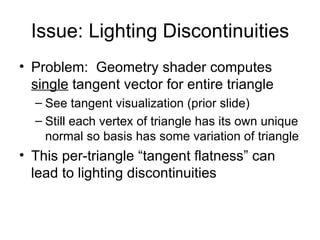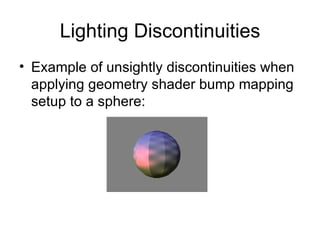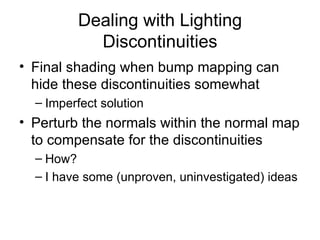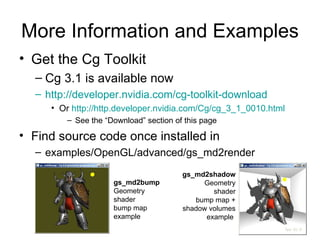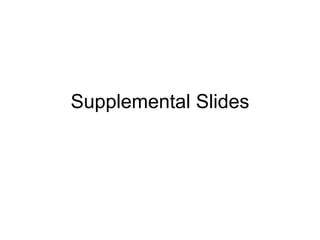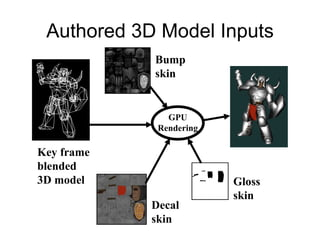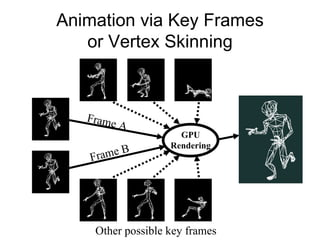1 of 24

### Geometry Shader-based Bump Mapping Setup

1. Geometry shader-based bump mapping setup Mark Kilgard NVIDIA Corporation February 5, 2007 Updated October 18, 2007
2. Geometry Shader in Cg TRIANGLE void md2bump_geometry( AttribArray < float4 > position : POSITION , AttribArray < float2 > texCoord : TEXCOORD0 , AttribArray < float3 > objPosition : TEXCOORD1 , AttribArray < float3 > objNormal : TEXCOORD2 , AttribArray < float3 > objView : TEXCOORD3 , AttribArray < float3 > objLight : TEXCOORD4 ) { float3 dXYZdU = objPosition - objPosition; float dSdU = texCoord.s - texCoord.s; float3 dXYZdV = objPosition - objPosition; float dSdV = texCoord.s - texCoord.s; float3 tangent = normalize (dSdV * dXYZdU - dSdU * dXYZdV); for ( int i=0; i<3; i++) { float3 normal = objNormal[i], binormal = cross (tangent,normal); float3x3 basis = float3x3 ( tangent , binormal, normal); float3 surfaceLightVector : TEXCOORD1 = mul (basis, objLight[i]); float3 surfaceViewVector : TEXCOORD2 = mul (basis, objView[i]); emitVertex (position[i], texCoord[i], surfaceLightVector, surfaceViewVector); } } warning: not tolerant of mirroring (see next version)
3. Canonical Triangle A B C A = ( u=0, v=0, x0, y0, z0, s0, t0 ) B = ( u=1, v=0, x1, y1, z1, s1, t1 ) C = ( u=0, v=1, x2, y2, z2, s2, t2 ) ∂ xyz / ∂u = (x1,y1,z1) - (x0,y0,z0) ∂ xyz / ∂v = (x2,y2,z2) - (x0,y0,z0) ∂ s / ∂u = s1 – s0 ∂ t / ∂v = t2 – t0 v u quotient rule for partial derivatives:
4. Forming an Object-to-Texture Space Basis assumes left-handed texture space provided by vertex shader
5. Transform Object-Space Vectors for Lighting to Texture-Space object-space texture-space light vector view vector
6. Compensating for Texture Mirroing If signed area in texture space “less than” zero, compute basis with negated tangent: 2x2 determinant
7. Example of Mirroring artist’s original decal derived height field RGB normal map generated from height field Visualization of 3D model’s signed area in texture space green =front-facing red =back-facing only half of face appears in decal
8. Consequence on Lighting from Accounting for Mirroring Bad: mirrored skull buckle and belt lighting wrong on left side (indents in) Correct lighting
9. Mirroring-tolerant Geometry Shader in Cg TRIANGLE void md2bump_geometry( AttribArray < float4 > position : POSITION , AttribArray < float2 > texCoord : TEXCOORD0 , AttribArray < float3 > objPosition : TEXCOORD1 , AttribArray < float3 > objNormal : TEXCOORD2 , AttribArray < float3 > objView : TEXCOORD3 , AttribArray < float3 > objLight : TEXCOORD4 ) { float3 dXYZdU = objPosition - objPosition; float dSdU = texCoord.s - texCoord.s; float3 dXYZdV = objPosition - objPosition; float dSdV = texCoord.s - texCoord.s; float3 tangent = normalize (dSdV * dXYZdU - dSdU * dXYZdV); float area = determinant ( float2x2 (dSTdV, dSTdU)); float3 orientedTangent = area >= 0 ? tangent : -tangent; for ( int i=0; i<3; i++) { float3 normal = objNormal[i], binormal = cross (tangent,normal); float3x3 basis = float3x3 (orientedTangent, binormal, normal); float3 surfaceLightVector : TEXCOORD1 = mul (basis, objLight[i]); float3 surfaceViewVector : TEXCOORD2 = mul (basis, objView[i]); emitVertex (position[i], texCoord[i], surfaceLightVector, surfaceViewVector); } } additional & changed code for mirroring
10. Discarding Triangles Significantly Back Facing w.r.t. the Light TRIANGLE void md2bump_geometry( AttribArray < float4 > position : POSITION , AttribArray < float2 > texCoord : TEXCOORD0 , AttribArray < float3 > objPosition : TEXCOORD1 , AttribArray < float3 > objNormal : TEXCOORD2 , AttribArray < float3 > objView : TEXCOORD3 , AttribArray < float3 > objLight : TEXCOORD4 ) { float3 dXYZdU = objPosition - objPosition; float dSdU = texCoord.s - texCoord.s; float3 dXYZdV = objPosition - objPosition; float dSdV = texCoord.s - texCoord.s; float3 tangent = normalize (dSdV * dXYZdU - dSdU * dXYZdV); float maxLightZ, maxLightThreshold = -0.3; for ( int i=0; i<3; i++) { float3 normal = objNormal[i], binormal = cross (tangent,normal); float3x3 basis = float3x3 ( tangent , binormal, normal); float3 surfaceLightVector : TEXCOORD1 = mul (basis, objLight[i]); maxLightZ = i==0 ? normalize(surfaceLightVector).z : max(maxLightZ, normalize(surfaceLightVector).z); float3 surfaceViewVector : TEXCOORD2 = mul (basis, objView[i]); if (i < 2 || maxLightZ > maxLightThreshold) emitVertex (position[i], texCoord[i], surfaceLightVector, surfaceViewVector); } }
11. Sans Per-vertex Normals
12. Geometry Shader in Cg Sans Normals TRIANGLE void md2bump_geometry_sans_normal( AttribArray < float4 > position : POSITION , AttribArray < float2 > texCoord : TEXCOORD0 , AttribArray < float3 > objPosition : TEXCOORD1 , // IGNORE per-vertex normal! AttribArray < float3 > objView : TEXCOORD3 , AttribArray < float3 > objLight : TEXCOORD4 ) { float3 dXYZdU = objPosition - objPosition; float2 dSTdU = texCoord - texCoord; float3 dXYZdV = objPosition - objPosition; float2 dSTdV = texCoord - texCoord; float3 tangent = normalize (dSTdV.s * dXYZdU - dSTdU.s * dXYZdV); float3 tangent2 = normalize (dSTdV.t * dXYZdU - dSTdU.t * dXYZdV); float area = determinant (float2x2(dSTdV, dSTdU)); tangent = area >= 0 ? tangent : -tangent; float3 normal = cross(tangent2,tangent); tangent2 = area >= 0 ? tangent2 : -tangent2; for ( int i=0; i<3; i++) { float3x3 basis = float3x3 (tangent, tangent2, normal); float3 surfaceLightVector : TEXCOORD1 = mul(basis, objLight[i]); float3 surfaceViewVector : TEXCOORD2 = mul(basis, objView[i]); emitVertex (position[i], texCoord[i], surfaceLightVector, surfaceViewVector); } }
13. Geometry shader setup with and without per-vertex normals Setup without per-vertex normals, relying on normalized gradients only; faceted look Setup with per-vertex normals; smoother lighting appearance
14. Supplemental Slides
15. Authored 3D Model Inputs Key frame blended 3D model Decal skin Bump skin Gloss skin GPU Rendering
16. Animation via Key Frames or Vertex Skinning GPU Rendering Frame A Frame B Other possible key frames